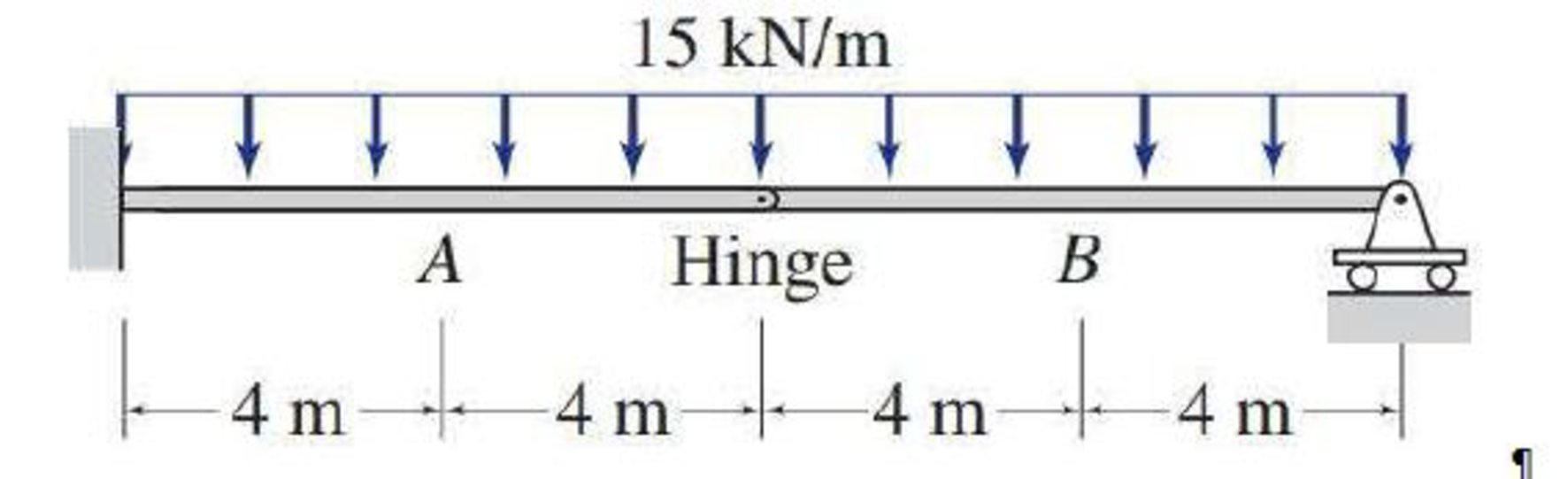# Determine the axial forces, shears, and bending moments at points A and B of the structure shown.

#### Solutions

Chapter
Section
Chapter 5, Problem 9P
Textbook Problem
33 views

## Determine the axial forces, shears, and bending moments at points A and B of the structure shown.To determine

Find the axial force, shear, and bending moment at points A and B of the beam.

### Explanation of Solution

Sign conversion:

Apply the sign convention for calculating the equations of equilibrium as below.

• For the horizontal forces equilibrium condition, take the force acting towards right side as positive (+) and the force acting towards left side as negative ().
• For the vertical forces equilibrium condition, take the upward force as positive (+) and downward force as negative ().
• For moment equilibrium condition, take the clockwise moment as negative and counter clockwise moment as positive.

Apply the following sign convention for calculating the axial forces, shear and bending moments.

• When the portion of the beam considered is left of the section, then the external force acting to the left are considered as positive.
• When the portion of the beam considered is right of the section, then the external force acting to the right are considered as positive.
• When the portion of the beam considered is left of the section, then the external force acting upward are considered as positive.
• When the portion of the beam considered is right of the section, then the external force acting downward are considered as positive.
• When the portion of the beam considered is left of the section, then the clockwise moments are considered as positive.
• When the portion of the beam considered is right of the section, then the counterclockwise moments are considered as positive.

Calculation:

Show the free-body diagram of the section from hinge to right end D as in Figure 1.

Find the vertical reaction at point D by taking moment about the hinge.

+Mhinge=0(15×8×82)+Dy(8)=0480+8Dy=0Dy=60kN

Show the free-body diagram of the entire beam as in Figure 2.

Find the horizontal reaction at point C by resolving the horizontal equilibrium.

+Fx=0Cx=0Cx=0

Find the moment at point C by taking moment about the point C.

+MC=0MC15×16×162+60(16)=0MC1920+960=0MC=960kN-m

Find the vertical reaction at point C by resolving the vertical equilibrium.

+Fy=0Cy15×16+60=0Cy=180kN

Pass the sections aa and bb at points A and B respectively.

Show the sections aa and bb as in Figure 3.

Consider section aa:

Consider the left side of the section aa for calculation of internal forces.

Show the free-body diagram of the left side of the section aa as in Figure 4.

### Still sussing out bartleby?

Check out a sample textbook solution.

See a sample solution

#### The Solution to Your Study Problems

Bartleby provides explanations to thousands of textbook problems written by our experts, many with advanced degrees!

Get Started

Find more solutions based on key concepts
Kinetic energy is another engineering quantity that is mass dependent. An object having a mass m and moving wit...

Engineering Fundamentals: An Introduction to Engineering (MindTap Course List)

Determine the magnitudes of the roller reactions at C and D. Neglect the weights of the members.

International Edition---engineering Mechanics: Statics, 4th Edition

A(n) ________ is a collaborative website that allows users to create, add to, modify, or delete the content via...

Enhanced Discovering Computers 2017 (Shelly Cashman Series) (MindTap Course List)

What is the purpose of the solid flux that covers the electrode?

Welding: Principles and Applications (MindTap Course List)

If your motherboard supports ECC DDR3 memory, can you substitute non-ECC DDR3 memory?

A+ Guide to Hardware (Standalone Book) (MindTap Course List)